CBSE Sample Papers for Class 9 Science Paper 1 are part of CBSE Sample Papers for Class 9 Science. Here we have given CBSE Sample Papers for Class 9 Science Paper 1.

## CBSE Sample Papers for Class 9 Science Paper 1

 Board CBSE Class IX Subject Science Sample Paper Set Paper 1 Category CBSE Sample Papers

Students who are going to appear for CBSE Class 9 Examinations are advised to practice the CBSE sample papers given here which is designed as per the latest Syllabus and marking scheme as prescribed by the CBSE is given here. Paper 1 of Solved CBSE Sample Paper for Class 9 Science is given below with free PDF download solutions.

Time Allowed : 3 Hours
Max. Marks: 80

General Instructions

• The question paper comprises of two Sections, A and B. You are to attempt both the sections.
• All questions are compulsory. However, an internal choice will be provided in two questions of 3 marks each and one question of five marks.
• All questions of Section A and all questions of Section B are to be attempted separately.
• Question numbers 1 to 2 in Section A are one-mark questions. These are to be answered in one word or in one sentence.
• Question numbers 3 to 5 in Section A are two-marks questions. These are to be answered in about 30 words each.
• Question numbers 6 to 15 in Section A are three-marks questions. These are to be answered in about 50 words each.
• Question numbers 16 to 21 in Section A are five-marks questions. These are to be answered in about 70 words each.
• Question numbers 22 to 27 in Section B are two-marks questions based on practical skills. These are to be answered in brief.

Questions
SECTION-A

Question 1.
What is the commercial unit of energy and state its equivalent value in joules.

Question 2.
Name the plastid involved in the conversion of green colour of tomato to red colour.

Question 3.
A man weighs 600 N on the surface of earth. What would be his mass and weight on the surface of moon? [ gearth = 10 m/s2 ]

Question 4.
A body is floating on the surface of a liquid. With the help of a diagram show the two forces acting on it that are responsible for its floatation. State the relationship between these two forces in this case.

Question 5.

1. Mention any two human activities which would be responsible for air pollution.
2. How is Earth’s atmosphere different from that of Venus and mars?

Question 6.
Distinguish between homogeneous and heterogeneous mixture. Classify the following mixtures as homogeneous and heterogeneous

1. Tincture of iodine
2. Smoke
3. Brass
4. Sugar solution

Question 7.
Derive graphically the equation for the position-time relation for an object travelling a distance ‘s’ in time ‘t’ under uniform acceleration.
OR
A stone dropped from a window reaches the ground in 0.5 seconds –
(i) Calculate its speed just before it hits the ground.
(ii) What is its average speed during 0.5 s?
(iii) Calculate the height of window from the ground.

Question 8.
(a) State two ways in which phloem is functionally different from xylem.
(b) Draw a neat diagram of a section of phloem and label its four parts.

Question 9.
State one difference between dugwells and tubewells. Explain any two fresh initiatives taken to increase the water available for agriculture.

Question 10.
(a) Which two factors bring about the loss of food grains during storage? Give one example for each.
(b) State any two control measures to be taken before grains are stored.
OR
Discuss with the help of suitable examples three ways in which microorganisms can enter into human body.

Question 11.

1. Write chemical formula of Aluminium Sulphate.
2. Give example each of a homodiatomic & homotetratomic molecule.

Question 12.

1. Identify two features possessed by all Chordates.
2. In which class would you place an organism which has
• a scaly exoskeleton and a bony endoskeleton
• a scaly exoskeleton and lay eggs outside water.

Question 13.
A flask contains 4.4 g of CO2 gas. Calculate
(a) How many moles of CO2 gas does it contain?
(b) How many molecules of CO2 gas are present in the sample.
(c) How many atoms of oxygen are present in the given sample.
[Atomic mass of C = 12 u, O = 16 u)

Question 14.
What is an Echo? State two conditions for echo to be heard. Bats cannot see still they catch their prey. Explain.

Question 15.

1. It was diagnosed that Preeti suffers from Japanese encephalitis, which organ of Preeti’s body is effected?
2. How are antibiotics effective in the treatment of some diseases?
3. Will they help in curing Preeti’s disease? Why?

Question 16.
Define ‘Power’ of a body.
State and define the S.I. unit of Power. Two children A and B both weighing 32 kg start climbing up a rope separately and reaches the height of 8m, ‘A’: takes 15 s and ‘B’ takes 20 s to reach that level. Calculate the amount of work done by A and B. Which of the two has more power. Show by calculation, [g = 10 m/s2]

Question 17.

1. Illustrate Rutherford’s experiment to explain the model of an atom.
2. Atomic number of an element is 17. Identify the element, write its electronic configuration & mention its valency.

Question 18.
The velocity time graph of a body is given as follows: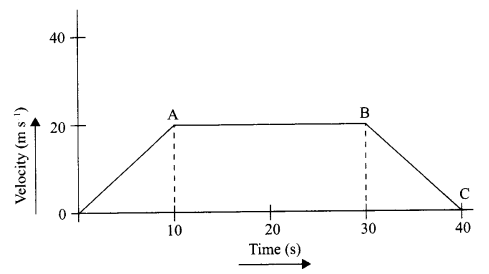1. State the kind of motion represented by OA; AB.
2. What is the velocity of the body after 10 s and after 40 s?
3. Calculate the retardation of the body.
4. Calculate the distance covered by the body between 10th and 30th second.

Question 19.
Apoultry farmer wants to increase his broiler production. Explain three management practices he must follow to enhance the yield.
In what way is the daily food requirement of broilers different from those of egg layers?

Question 20.
Distinguish solids, liquids and gases in a tabular form under the following characteristics:

1. Rigidity
2. Compressibility
3. Inter-particle forces of attraction
4. Inter-particle spaces
5. Kinetic energy of particles

Question 21.
(a) Draw a neat and labelled diagram of the apparatus used to separate components of blue- black ink. Name the process and state the principle involved.
(b) Identify the physical and chemical changes from the following:

1. Burning of magnesium in air
2. Tarnishing of silver spoon
3. Sublimation of iodine
4. Electrolysis of water

OR
(a) Write down the chemical formulae of the following compounds:

1. Magnesium Nitride
2. Calcium Nitrate
3. Sodium Carbonate

(b) Find out the number of atoms present in:

1. PO3-4 ion
2. H2SO3 molecule

SECTION-B

Question 22.
The spring balance shown here is used to measure the mass of a given solid. Calculate the mass of the solid.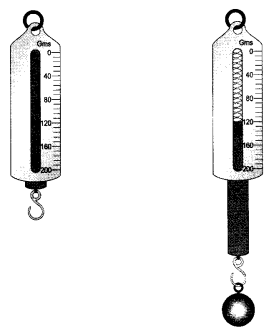Question 23.
Waves in a slinky travelles in to and for motion as shown; calculate the velocity of the wave.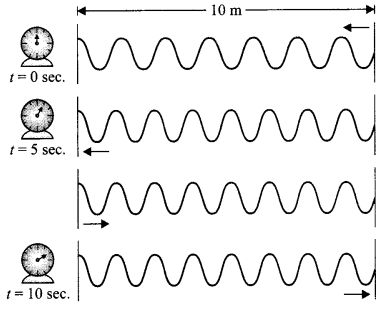Question 24.
The diagram shows life cycle of a mosquito, the missing stages marked as (i) and (ii) areQuestion 25.
Give the chemical formula of sugar and alum.

Question 26.
How will you calculate the minimum distance for an echo?

Question 27.
A measuring cylinder has 5 mark between 10 mL to 20 mL. What is its least count?

SECTION : A

Kilowatt hour
1 kilowatt hour = 3.6 × 106 joules

Chromoplast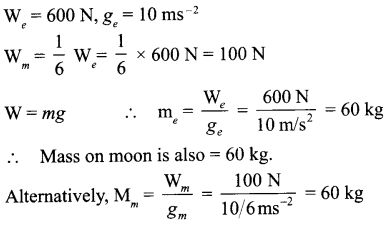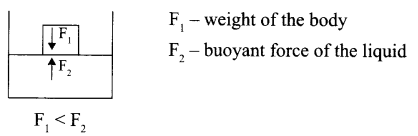• Increased use of vehicles run by fossil fuels increases the gaseous air pollutants.
• Factory outlets, low efficiency engines throw exhaust gases in the air.
1. In Earth’s atmosphere, CO2 is 0.03% of the total of all gases and in the atmosphere of Mars and Venus it is 95 – 97% of all the gases.

 Homogeneous Mixture Heterogeneous Mixture (i) Uniform composition throughout its mass. Does not have a uniform composition throughout its mass. (ii) No visible boundaries of separation between the constituents. Visible boundaries of separation between the constituents.

1. Tincture of iodine – Heterogeneous
2. Smoke – Heterogeneous
3. Brass – Heterogeneous
4. Sugar solution – Heterogeneous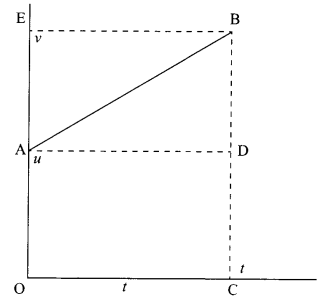Distance travelled by the object = Area of graph under the curve
= area of trapezium OABC
= area of rectangle OADC + area of triangle ABD(a)

1. Phloem transports food from leaves to other parts of the plant body unlike xylem, which transports water and minerals from roots to other parts of the plant.
2. Phloem unlike xylem allows movement of materials in both the directions, i.e. both towards the root and also away from the root.

(b)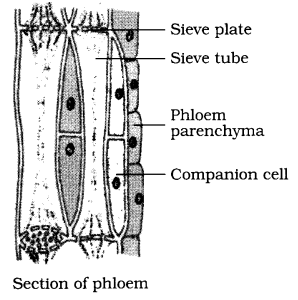1. Dugwell – Water is collected from water bearing strata, manually using a bucket.
Tubewell – Water is collected from deeper strata using a handle present on it.
2. Rain water harvesting and Watershed management are the two initiatives taken to increase the water supply for agriculture. These initiatives involve building small check dams to stop the rain water to flow away.

(a)

• Biotic factors – Insects/ rodents/ fungi/ mites (any one)
• Abiotic factors – Inappropriate temperature/ Inappropriate moisture

(b) Any two of the following measures –

• strict cleaning of product before storage
• proper sundrying and then drying in shade
• Fumigation
• systematic management of warehouses

OR

• Through cuts and wounds (Tetanus)
• Through contaminated food and water (cholera)
• Through sexual contact (AIDS, Syphillis)
• Through air (TB, Pneumonia)
• Through direct skin contact (Ring worm) (any three)

1. Aluminium sulphate:2. Homo-diatomic molecule – O2 /H2/N2
Homo-tetratomic molecule – P4

1. Presence of notochord/ dorsal nerve chord/ paired gill pouches/ have a bilaterally symmetrical body/ are triploblastic are coelomate. (any two)

• Class Pisces
• Class Reptilia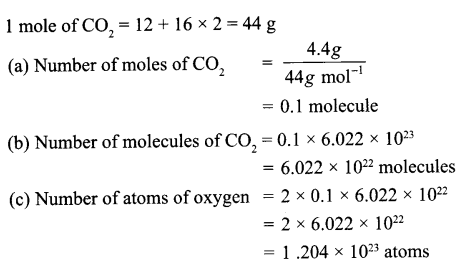1. Echo – sound heard after reflection from an obstacle.
2. Conditions –
• time interval between the original sound and reflected sound must be at least 0.1 s
• minimum distance of the obstacle from the source of sound must be 17.2 m at 22°C. = 17 – 18 m at room temperature.
3. Bats produce ultrasonic waves. These signals are received by them after reflection from the prey and interpreted by their brain accordingly.

1. Brain
2. They block the biochemical pathways. As a result of this the bacteria are unable to make cell walls and so they die.
3. No, Japanese encephalitis is a viral disease.

• Power is the rate of doing work/rate of transfer of energy.
• S.I. unit of power is watt
• I watt is the power of an agent which does work at the rate of 1 joule per second/ power
of an agent when the rate of consumption of energy is 1 joule per second.

For A:
W = mgh
= 32 kg × 10 m/s2 × 8 m
W = 2560 J
Both have same mass and climb the same height.
∴ Work done by B = 2560 J
P = $$\frac { 2560J }{ 15S }$$ = 170.7 W
P = $$\frac { 2560J }{ 20S }$$ = 128 W
∴ A has more power

1. Rutherford’s scattering experiment: He selected a sheet of very thin gold foil to be bombarded with fast moving a-particles – which were doubly positively charged ‘He’ ions.
The fast moving a-particles have a considerable amount of kinetic energy to hit the gold atoms
Observations:

• Most of the a-particles passed straight through the foil.
• One out of 20,000 particles appeared to rebound.

Inference:

• There is a positive centre inside the atom called nucleus.
• The electrons revolve around the nucleus in well defined orbits.
• The size of the nucleus is very small as compared to the size of the atom.

2. Atomic number is 17
Element is chlorine
Electronic configuration – 2, 8, 7; valency – 1

1. OA – uniform acceleration, AB – zero acceleration/constant velocity.
2. 20 ms-1; zero/body comes to rest.
3. Retardation = $$\frac { { (0-20)ms }^{ -1 } }{ (40-30)s }$$ m s-1 = -10 m s-1
4. Distance between 10th and 30th second = area of shaded figure
= (30 – 10)s × 20 m s-1
= 20 s × 20 m s-1
= 400 m1. Maintenance of temperature
2. Provision of hygienic conditions in housing and poultry feed
3. Prevention and control of diseases and pests
The broiler’s food must be rich in protein, fat, vitamin A and vitamin K, as the food should help in good growth rate, to develop more muscles and to maintain feathering and carcass quality.

 Characteristics Solid Liquid Gas Rigidity Rigid – maintains hardness and shape Fluid – flow easily Fluid – capable of infinite expansion Compressibility Almost incompressible Relatively incompressible Highly compressible Inter-particle forces of attraction Strongest (keep the particles together) Comparatively Weaker (but keep the particles within the bulk of liquid Extremely weak particles are free to move in all directions Inter-particle spaces Nearly negligible Intermediate Very large Kinetic energy of particles Very low Low HighProcess: Chromatography
Principle: The coloured component that is more soluble in water rises faster and in this way, the colours of different dyes present in blue-black ink get separated.
(b)

1. Chemical change
2. Chemical change
3. Physical change
4. Chemical change

OR
(a) The chemical formulae of the compounds are as follows:

1. Magnesium Nitride – Mg3N2
2. Calcium Nitride – Ca(NO3)2
3. Sodium Carbonate – Na2CO3

(b)

1. The number of atoms in PO3-4 i is 5.
2. The number of atoms in H2SO3 molecule is 6.

SECTION : B

Initial reading of the spring balance = 10 g
Final reading of the spring balance = 122.5 g
Final reading = 122.5- 10= 112.5 g

velocity = $$\frac { Distance }{ time }$$ ; $$\frac { 20m }{ 10 }$$ = 2 m/s

• Larva
• Pupa

• Sugar – Sucrose → C12H22O11
• Alum – K2SO4 . Al2(SO4)3 . 24H2O

Speed of sound = $$\frac { Distance }{ Time }$$
344 m/s = $$\frac { Distance }{ 1.0s }$$